GFG App
Open AppBrowser
Continue

# Class 10 RD Sharma Solutions – Chapter 11 Constructions – Exercise 11.2 | Set 1

### Question 1. Construct a triangle of sides 4 cm, 5 cm, and 6 cm and then a triangle similar to it whose sides are (2/3) of the corresponding sides of it.

Solution:

Follow these steps for the construction:

Step 1: Construct a line segment BC of 5 cm.

Step 2: From the centre B take the radius of 4 cm and from the centre C take the radius 6 cm, construct arcs bisecting each other at the point A.

Step 3: Connect the lines AB and AC.

After that we will have ABC as the triangle.

Step 4: Construct a ray BX creating an acute angle with the line BC and break off 3 equal parts creating

BB1 = B1B2 = B2B3.

Step 5: Further connect B3C.

Step 6: Construct B’C’ parallel to B3C and C’A’ parallel to CA

Therefore,

We have the required triangle ΔA’BC’.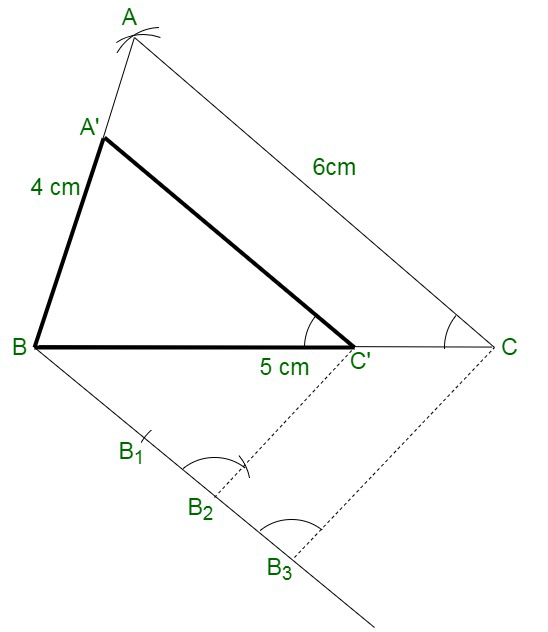### Question 2. Construct a triangle similar to a given ΔABC such that each of its sides is (5/7)th of the corresponding sides of ΔABC. It is given that AB = 5 cm, BC = 7 cm and ∠ABC = 50°.

Solution:

Follow these steps for the construction:

Step 1: Construct a line segment BC of 7 cm.

Step 2: Construct a ray BX  an angle of 50° and break off BA = 5 cm.

Step 3: Connect AC.

After that we have ABC is the triangle.

Step 4: Construct a ray BY creating an acute angle with BC and break off 7 equal parts creating

BB = B1B2 = B2B3 = B3B4 = B4Bs = B5B6 = B6B7

Step 5: Now connect B7 and C

Step 6: Construct B5C’ parallel to B7C and C’A’ parallel to CA.

Therefore,

We have the required triangle ΔA’BC’.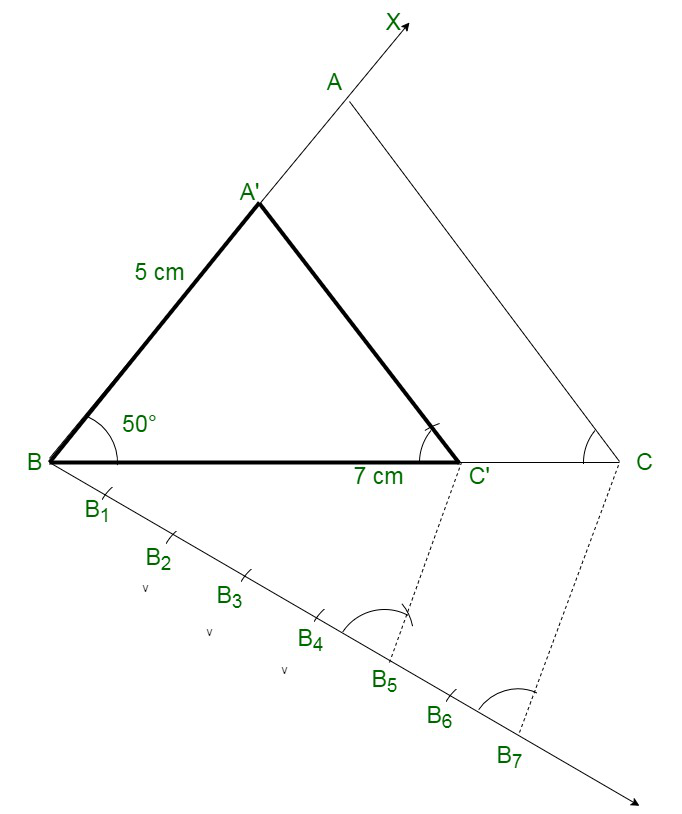### Question 3. Construct a triangle similar to a given ΔABC such that each of its sides is (2/3)rd of the corresponding sides of ΔABC. It is given that BC = 6 cm, ∠B = 50° and ∠C = 60°.

Solution:

Follow these steps for the construction:

Step 1: Construct a line segment BC of 6 cm.

Step 2: Construct a ray BX creating an angle of 50° and CY creating 60° Along BC which bisect each other at A. After that, ABC is the triangle.

Step 3: From B, Construct one more ray BZ creating an acute angle below BC and intersect 3 equal parts, creating BB1 = B1B2 = B2B2.

Step 4: Connect B3C.

Step 5: From B2, Construct B2C’ parallel to B3C and C’A’ parallel to CA.

Therefore,

We have the required triangle ΔA’BC’.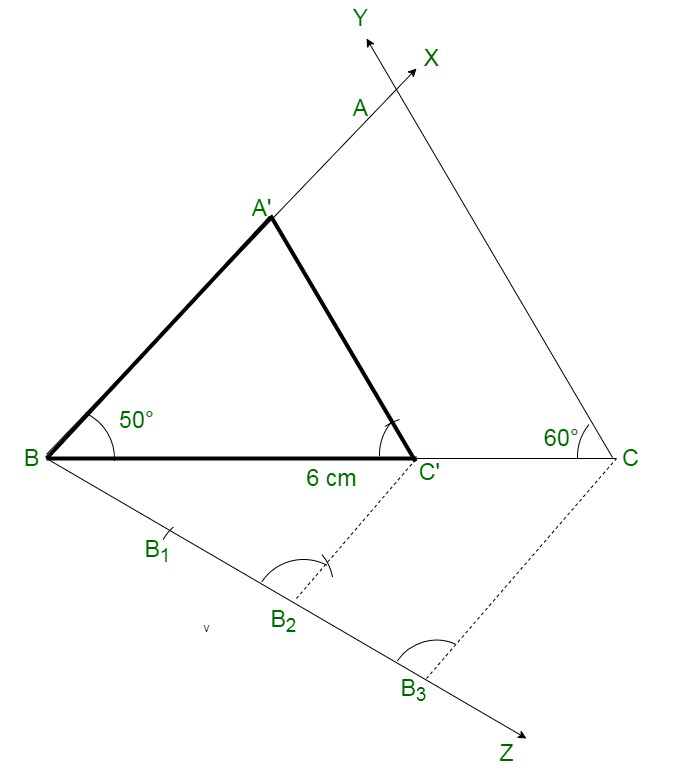### Question 4. Construct a ΔABC in which BC = 6 cm, AB = 4 cm and AC = 5 cm. Draw a triangle similar to ΔABC with its sides equal to 3/4th of the corresponding sides of ΔABC.

Solution:

Follow these steps for the construction:

Step 1: Construct a line segment BC of 6 cm.

Step 2: Along centre B and radius 4 cm and Along centre C and radius 5 cm,

Construct arcs’ bisecting each other at A.

Step 3: Connect AB and AC.

After that ABC is the triangle,

Step 4: Construct a ray BX creating an acute angle along BC and break off 4 equal parts creating BB1=  B1B2 = B2B3 = B3B4.

Step 5: Connect B4 and C.

Step 6: From B3C Construct C3C’ parallel to B4C and from C’,

Step 7: Construct C’A’ parallel to CA.

Therefore,

We have the required triangle ΔA’BC’.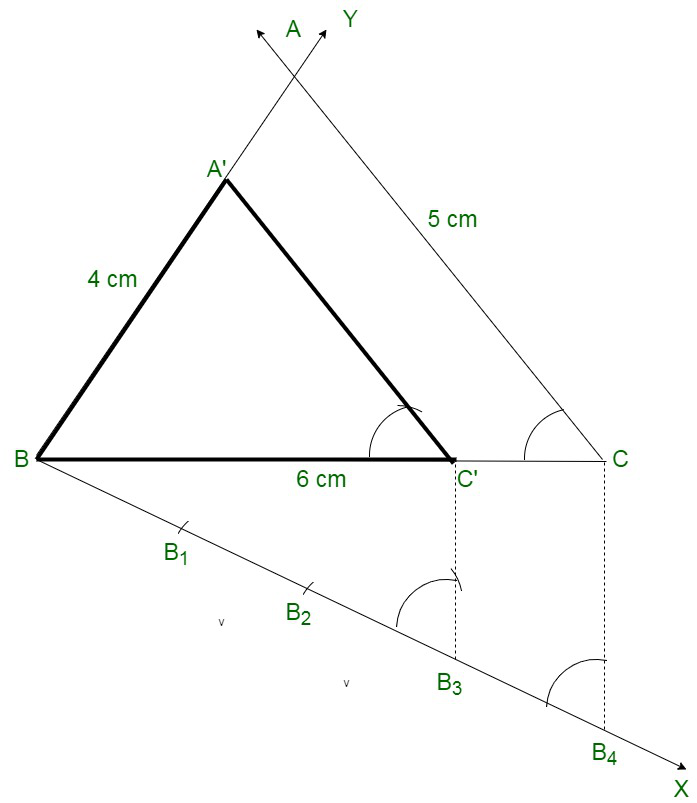### Question 5. Construct a triangle Along sides 5 cm, 6 cm, and 7 cm and then another triangle whose sides are (7/5)th of the corresponding sides of the first triangle. Give the justification of the construction.

Solution:

Follow these steps for the construction:

Step 1: Construct a line segment BC of 5 cm.

Step 2: Along centre B and radius 6 cm and Along centre C and radius 7 cm,

Construct arcs bisecting each other at A.

Step 3: Connect AB and AC.

After that ABC is the triangle.

Step 4: Construct a ray BX creating an acute angle along BC and break off 7 equal parts creating

BB1 = B1B2 = B2B3 = B3B4 = B4B5 = B5B6 = B6B7.

Step 5: Connect B5 and C.

Step 6: From B7, Construct B7C’ parallel to B5C and C’A’ parallel CA.

Therefore,

We have the required triangle ΔA’BC’.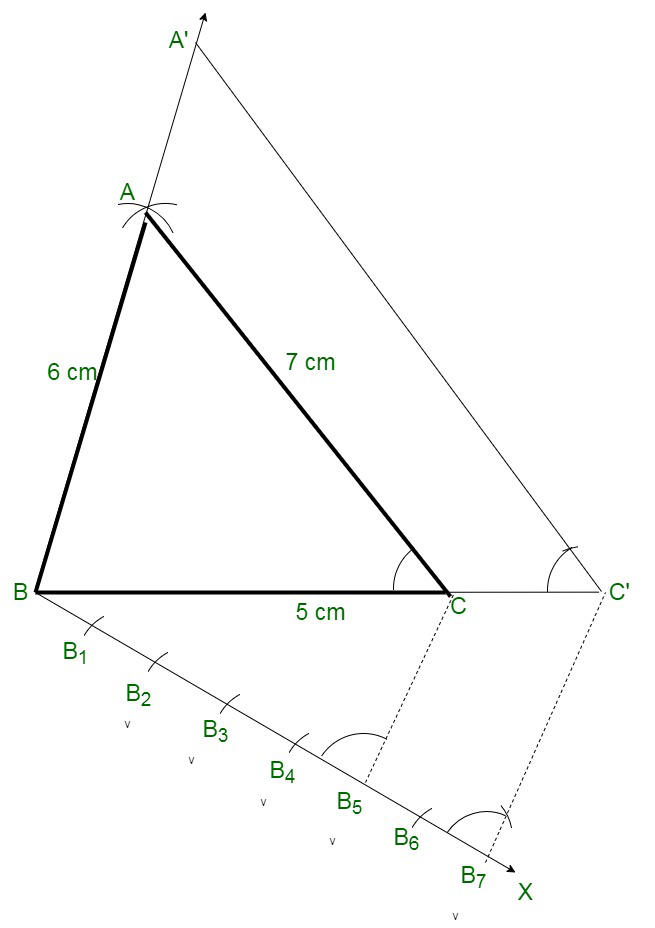### Question 6. Construct a right triangle ABC in which AC = AB = 4.5 cm and ∠A = 90°. Draw a triangle similar to ΔABC with its sides equal to (5/4)th of the corresponding sides of ΔABC.

Solution:

Follow these steps for the construction:

Step 1: Construct a line segment AB of 4.5 cm.

Step 2: At A, Construct a ray AX perpendicular to AB and break off AC = AB = 4.5 cm.

Step 3: Connect BC.

After that ABC is the triangle.

Step 4: Construct a ray AY creating an acute angle along AB and break off 5 equal parts creating

AA1 = A1A2 = A2A3 = A3A4 = A4A5

Step 5: Connect A4 and B.

Step 6: From 45, Construct 45B’ parallel to  A4B and  B’C’ parallel to BC.

Therefore,

We have the required triangle ΔAB’C’.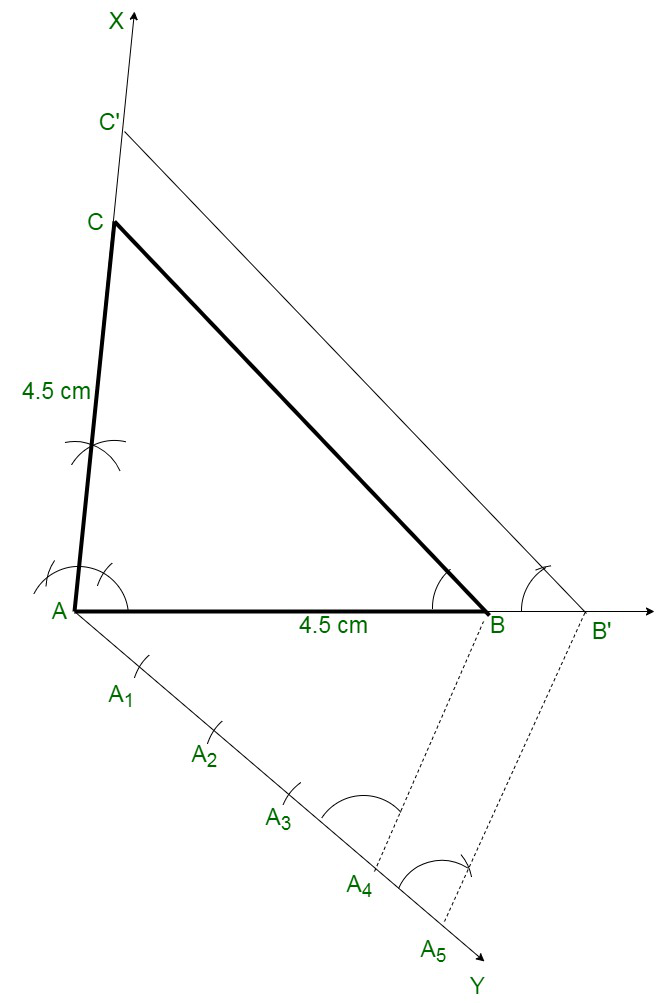### Question 7. Construct a right triangle in which the sides (other than hypotenuse) are of lengths 5 cm and 4 cm. Then construct another triangle whose sides are 5/3 times the corresponding sides of the given triangle.

Solution:

Follow these steps for the construction:

Step 1: Construct a line segment BC of 5 cm.

Step 2: At B, Construct perpendicular BX and break off BA = 4 cm.

Step 3: Connect AC ,

After that ABC is the triangle

Step 4: Construct a ray BY creating an acute angle along BC, and break off 5 equal parts creating

BB1 = B1B2 = B2B3 = B3B4 = B4B5.

Step 5: Connect B3 and C.

Step 6: From B5, Construct B5C’ parallel to B3C and C’A’ parallel to CA.

Therefore,

We have the required triangle ΔA’BC’.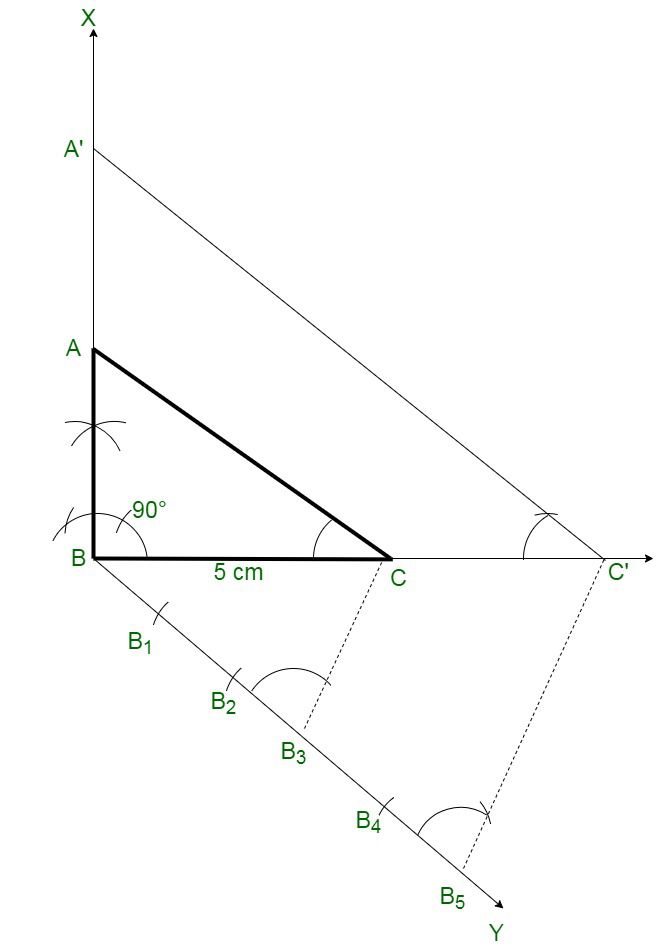### Question 8. Construct an isosceles triangle whose base is 8 cm and altitude 4 cm and then another triangle whose sides are 3/2 times the corresponding sides of the isosceles triangle.

Solution:

Follow these steps for the construction:

Step 1: Construct a line segment BC of 8 cm.

Step 2: Construct its perpendicular bisector DX and break off DA = 4 cm.

Step 3: Connect AB and AC.

After that ABC is the triangle.

Step 4: Construct a ray DY creating an acute angle along OA and break off 3 equal parts creating

DD1 = D1D2 = D2D3 = D3D4.

Step 4: Connect D2.

Step 5: Construct D3A’ parallel to D2A and A’B’ parallel to AB meeting BC at C’ and B’ respectively.

Therefore,

We have the required triangle ΔB’A’C’.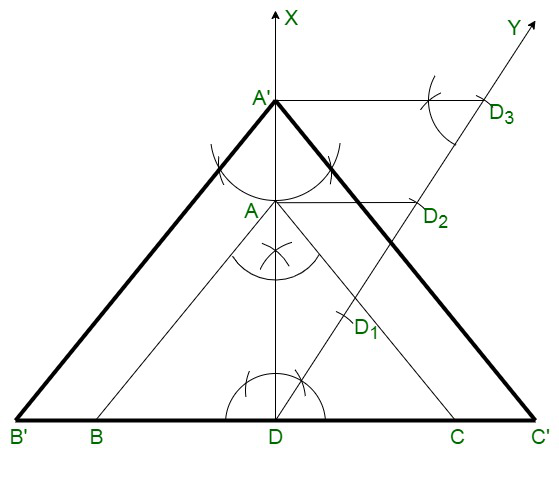### Question 9. Draw a ΔABC with BC = 6 cm, AB = 5 cm and ∠ABC = 60°. Then construct a triangle whose sides are (3/4)th of the corresponding sides of the ΔABC.

Solution:

Follow these steps for the construction:

Step 1: Construct a line segment BC of 6 cm.

Step 2: At B, Construct a ray BX creating an angle of 60° Along BC and break off BA of 5 cm.

Step 3: Connect AC. After that ABC is the triangle.

Step 4: Construct a ray BY creating an acute angle along BC and break off 4 equal parts creating

BB1= B1B2 = B2B3=B3B4.

Step 5: Connect B4 and C.

Step 6: From B3, Construct B3C’ parallel to B4C and C’A’ parallel to CA.

Therefore,

We have the required triangle ΔA’BC’.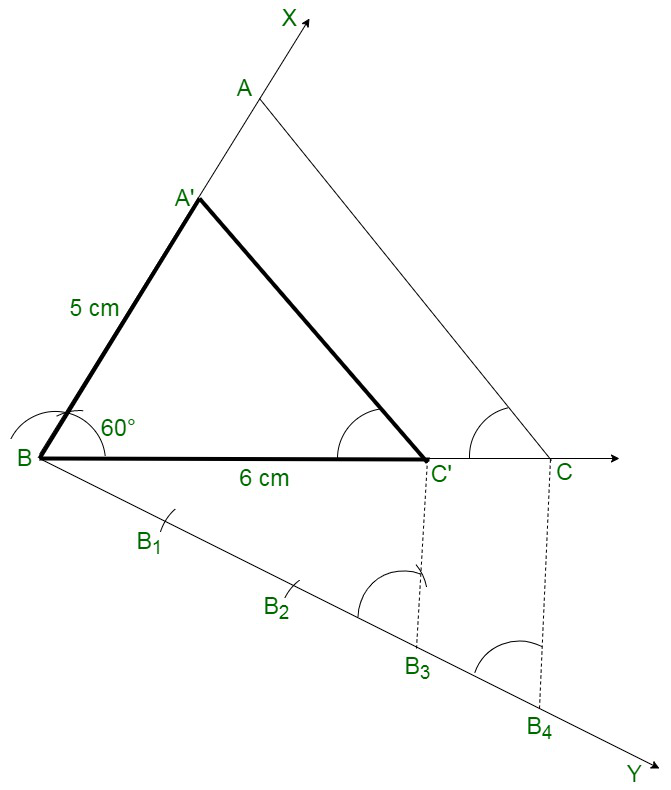### Question 10. Construct a triangle similar to ΔABC in which AB = 4.6 cm, BC = 5.1 cm, ∠A = 60° Along scale factor 4 : 5.

Solution:

Follow these steps for the construction:

Step 1: Construct a line segment AB of 4.6 cm.

Step 2: At A, Construct a ray AX creating an angle of 60°.

Step 3: Along centre B and radius 5.1 cm.

Construct an arc bisecting AX at C.

Step 4: Connect BC.

After that ABC is the triangle.

Step 5: From A, Construct a ray AX creating an acute angle along AB and break off 5 equal parts creating

AA1 = A1A2 = A2A3 = A3A4=A4A5.

Step 6: Connect A4 and B.

Step 7: From A5, ConstructA5B’ parallel to A4B and B’C’ parallel to BC.

Therefore,

We have the required triangle ΔC’AB’.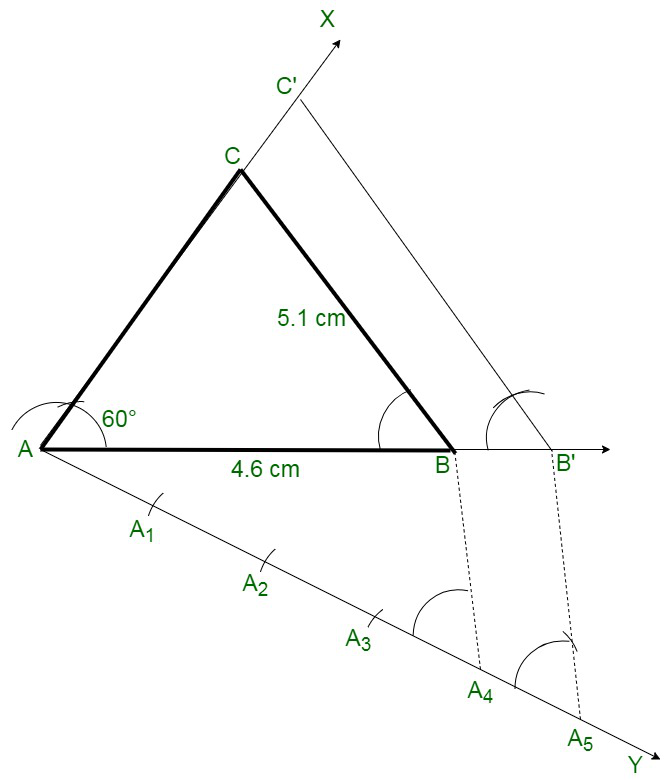My Personal Notes arrow_drop_up